# How to Count Unique Numeric Values in a Range in Excel

This post will guide you how to count unique numeric values in the given range in Excel 2013/2016 or Excel office 365. How do I count the unique numeric values in a list of data with some duplicate values using a formula in Excel. And you can use a formula that uses the SUM function together with the FREQUENCY function to count unique values in a range.

## COUNT Unique Numeric Values

Assuming you have a list of data range B1:B6 in your worksheet and you wish to count the number of unique numeric values using a formula. And the below introduction will show you the ways to achieve the result. The generic formula is like this:

=SUM(–(FREQUENCY(B1:B6,B1:B6)>0))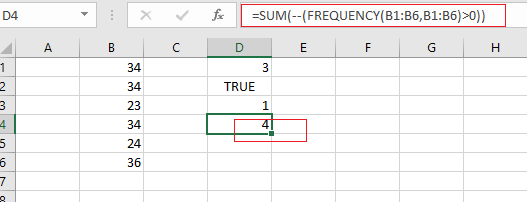Note: if you are working in Excel 365 version, and you can use a dedicated function called UNIQUE to count unique values. It can be used to count unique values including numeric or text values.

Let’s See How This Formula Works:

=FREUQENCY(B1:B6,B1:B6)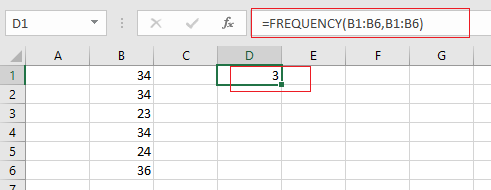The FREQUENCY function can be used to count each of numeric value in the range B1:B6, and returns an array result, like this:

{3;0;1;0;1;1;0}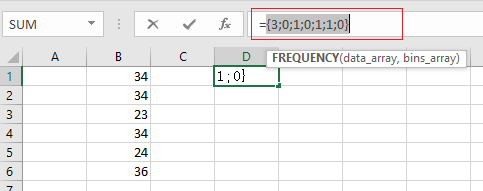From the above array result, you can see that the FREQUENCY function returns zero for any numbers that appear more that once in the data array, which is why values are zero once a number has been counted.

= FREQUENCY(B1:B6,B1:B6)>0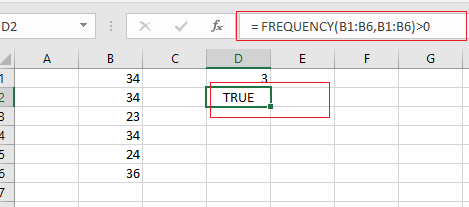You need to test each values that is greater than zero. The array result is like this:

{TRUE;FALSE;TRUE;FALSE;TRUE;TRUE;FALSE}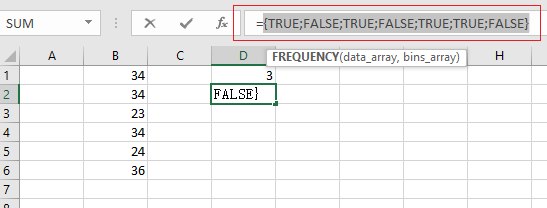Echo “TRUE” value represents a unique numeric value in the range B1:B6, and you still need to add up the TRUE values with the SUM function.

= –(FREQUENCY(B1:B6,B1:B6)>0)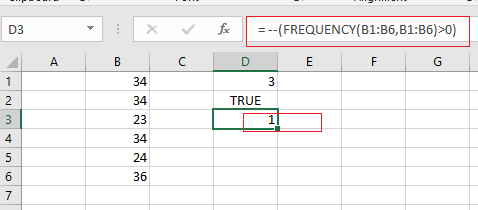The SUM function can be used to add up logic values in an array, and you need to coerce those values into 1 or 0 by the double negative operator

{1;0;1;0;1;1;0}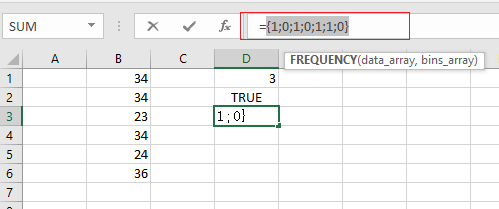Finally, you can use the SUM function to adds these values up in the above result array and return the total number is 4. Also you can use the SUMPRODUCT function to sum the values in the given array.

You can also use another way to count unique numeric values that is to use the COUNTIF function on the larger data list.

### Related Functions

• Excel SUM function
The Excel SUM function will adds all numbers in a range of cells and returns the sum of these values. You can add individual values, cell references or ranges in excel.The syntax of the SUM function is as below:= SUM(number1,[number2],…)…
• Excel FREQUENCY function
TThe Excel FREQUENCY function calculates how often values occur within a range of values. And it will return a vertical array of numbers.The syntax of the FREQUENCY function is as below:= FREQUENCY (data_array, bins_array)…
• Excel SUMPRODUCT function
The Excel SUMPRODUCT function multiplies corresponding components in the given one or more arrays or ranges, and returns the sum of those products.The syntax of the SUMPRODUCT function is as below:= SUMPRODUCT (array1,[array2],…)…
• Excel COUNTIF function
The Excel COUNTIF function will count the number of cells in a range that meet a given criteria. This function can be used to count the different kinds of cells with number, date, text values, blank, non-blanks, or containing specific characters.etc.= COUNTIF (range, criteria)…